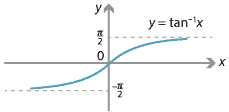## Answers to exercises

##### Exercise 1
1. Assume that $$f \colon \mathbb{R} \to \mathbb{R}$$ is both even and odd. Let $$x \in \mathbb{R}$$. Since $$f$$ is even, we have $$f(-x) = f(x)$$, and since $$f$$ is odd, we have $$f(-x) = -f(x)$$. Therefore $$f(x) = -f(x)$$, which implies that $$f(x)=0$$. So $$f$$ is constant zero.
2. Consider a polynomial $$f(x) = a_0 + a_1x + a_2x^2 + \dots + a_nx^n$$. We will assume that $$n$$ is even. (The case that $$n$$ is odd is similar.) Take \begin{align*} g(x) &= a_0 + a_2x^2 + a_4x^4 + \dots + a_nx^n \\ h(x) &= a_1x + a_3x^3 + a_5x^5 + \dots + a_{n-1}x^{n-1}. \end{align*} Then $$f(x) = g(x)+h(x)$$, with $$g(x)$$ an even function and $$h(x)$$ an odd function.
3. Suppose $f(x) = g(x)+h(x)\qquad\qquad (1)$ such that $$g(x)$$ is odd and $$h(x)$$ is even. Then \begin{align*} f(-x) &= g(-x)+h(-x) \\ &= -g(x)+h(x). \qquad (2) \end{align*} Adding equations (1) and (2) gives $$f(x) + f(-x) = 2h(x)$$, and we obtain $h(x) = \dfrac{f(x)+f(-x)}{2} \qquad\text{and}\qquad g(x)=\dfrac{f(x)-f(-x)}{2}.$ This gives us the uniqueness of $$g(x)$$ and $$h(x)$$. We can easily check that the function $$g(x)$$ defined this way actually is an odd function, and similarly that $$h(x)$$ actually is an even function.
##### Exercise 2
1. If $$f_1$$ and $$f_2$$ are odd functions, then $(f_1+f_2)(-x) = f_1(-x)+f_2(-x) = -f_1(x)-f_2(x) = -(f_1+f_2)(x),$ so $$f_1+f_2$$ is odd. If $$g_1$$ and $$g_2$$ are even functions, then $(g_1+g_2)(-x)=g_1(-x)+g_2(-x)=g_1(x)+g_2(x)=(g_1+g_2)(x),$ so $$g_1+g_2$$ is even.
2. Suppose $$f_1$$ and $$f_2$$ are even, and $$g_1$$ and $$g_2$$ are odd. If $$h = f_1f_2$$, then $h(-x) = f_1(-x)\,f_2(-x) = f_1(x)\,f_2(x) = h(x),$ so $$h$$ is even. If $$j = g_1g_2$$, then $j(-x) = g_1(-x)\,g_2(-x) = -g_1(x)\times -g_2(x) = g_1(x)\,g_2(x) = j(x),$ so $$j$$ is even. If $$k = f_1g_1$$, then $k(-x) = f_1(-x)\,g_1(-x) = f_1(x)\times -g_1(x) = -f_1(x)\,g_1(x) = -k(x),$ so $$k$$ is odd.
3. Let $$f \colon D \to \mathbb{R}$$ with $$f(a) \neq 0$$, for some $$a \in D$$, and let $$g \colon D \to \mathbb{R}$$ with $$g(b) \neq 0$$, for some $$b \in D$$. Assume that $$f$$ is odd and $$g$$ is even. Then \begin{alignat*}{2} (f+g)(-a) &= f(-a) + g(-a) \\ &= -f(a) + g(a) &\qquad&\text{since } f \text{ is odd and } g \text{ is even} \\ &\neq f(a) + g(a) &&\text{since } f(a) \neq 0 \\ &= (f+g)(a). \end{alignat*} So $$f+g$$ is not even. Similarly, we can use $$b$$ to show that $$f+g$$ is not odd.
##### Exercise 3

Let $$y = x^2+4x+10 = (x+2)^2+6$$. To map $$y=x^2$$ to $$y=(x+2)^2+6$$, translate it 2 units to the left and 6 units up.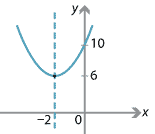##### Exercise 4
1. First assume that $$f$$ is even. Then $$f(-x)=f(x)$$, for all $$x$$ in the domain. So reflection in the $$y$$-axis maps $$y=f(x)$$ to $$y=f(-x)=f(x)$$.

For the converse, assume that $$y=f(x)$$ is mapped to itself under reflection in the $$y$$-axis. Then $$y=f(-x)$$ is $$y=f(x)$$. Thus $$f(-x)=f(x)$$, for all $$x$$ in the domain of $$f$$, and so $$f$$ is even.

2. Note that $$y=f(x)$$ is mapped to $$y=-f(x)$$ by reflection in the $$x$$-axis, which is then mapped to $$y=-f(-x)$$ by reflection in the $$y$$-axis. If the resulting graph is $$y=f(x)$$, then $$f(x)=-f(-x)$$, for all $$x$$ in the domain of $$f$$, and so $$f$$ is odd. Check the converse.

##### Exercise 5
1. Let $$g(x) = x-7$$. Then \begin{align*} f(g(x)) &= f(x-7) = x-7+7 = x \\ g(f(x)) &= g(x+7) = x+7-7 = x, \end{align*}

for all $$x$$. Hence, $$f$$ and $$g$$ are inverses of each other.

2. Let $$g(x) = \dfrac{x-5}{4}$$. Then \begin{align*} f(g(x)) &= f\Bigl(\dfrac{x-5}{4}\Bigr) = 4\times \Bigl(\dfrac{x-5}{4}\Bigr) + 5 = x \\ g(f(x)) &= g(4x+5) = \dfrac{4x+5-5}{4} = x. \end{align*}

Thus $$f$$ and $$g$$ are inverses of each other.

##### Exercise 6
1. Note that $$\mathrm{domain}(f) = \mathrm{range}(f) = \mathbb{R}$$ and $$\mathrm{domain}(g) = \mathrm{range}(g) = \mathbb{R}$$. We have \begin{align*} (f\circ g)(x) &= f(g(x)) = f(x^{\dfrac{1}{5}}) = (x^{\dfrac{1}{5}})^5 = x \\ (g\circ f)(x) &= g(f(x)) = g(x^5) = (x^5)^{\dfrac{1}{5}} = x, \end{align*} for all $$x \in \mathbb{R}$$. Thus $$f \circ g = \mathrm{id}$$ and $$g\circ f = \mathrm{id}$$, as required.
2. Let $$f(x) = x^3+2$$. We have $$x^3=f(x)-2$$ and so $$x=\sqrt{f(x)-2}$$. Take $$g(x) = \sqrt{x-2}$$. This gives $$f\circ g = \mathrm{id}$$ and $$g\circ f = \mathrm{id}$$, as required.
##### Exercise 7

The function $$f(x)=3x+2$$ has graph $$y=3x+2$$. To find the inverse, we swap $$x$$ and $$y$$. This gives

\begin{align*} x &= 3y+2 \\ y &= \dfrac{x-2}{3}. \end{align*}

Thus the inverse is given by $$f^{-1}(x) = \dfrac{x-2}{3}$$. To find where the two lines meet, we solve $$f(x) = f^{-1}(x)$$. This gives

\begin{align*} 3x+2 &= \dfrac{x-2}{3} \\ 9x+6 &= x-2 \\ x &= -1. \end{align*}

Substituting $$x = -1$$ into $$y = 3x+2$$ yields $$y=-1$$. Hence, they meet on the line $$y=x$$.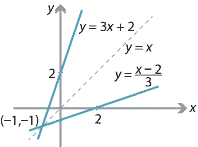##### Exercise 8
1. Consider the graph of $$y = \cos x$$.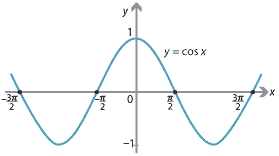We must restrict the domain of $$\cos x$$ so that, for each $$c$$ with $$-1\leq c\leq 1$$, there is exactly one value of $$x$$ such that $$\cos x=c$$. We restrict the domain of $$\cos x$$ to the interval $$[0,\pi]$$. This gives us a strictly decreasing function with domain $$[0,\pi]$$ and range $$[-1,1]$$. The inverse function $$\cos^{-1} x$$ has domain $$[-1,1]$$ and range $$[0,\pi]$$.
So $$\cos^{-1} a = b$$ if and only if $$\cos b = a$$, for $$a \in [-1,1]$$, $$b\in [0,\pi]$$.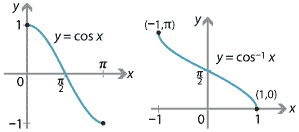2. Consider the graph of $$y=\tan x$$.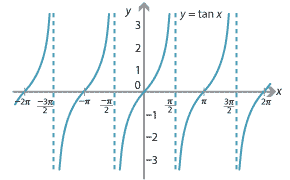We restrict the domain of $$\tan x$$ to the interval $$(-\dfrac{\pi}{2},\dfrac{\pi}{2})$$. This gives us a strictly increasing function with domain $$(-\dfrac{\pi}{2},\dfrac{\pi}{2})$$ and range $$\mathbb{R}$$. The inverse function $$\tan^{-1} x$$ has domain $$\mathbb{R}$$ and range $$(-\dfrac{\pi}{2},\dfrac{\pi}{2})$$. So $$\tan^{-1} a = b$$ if and only if $$\tan b = a$$, for $$a \in \mathbb{R}$$, $$b\in (-\dfrac{\pi}{2},\dfrac{\pi}{2})$$.